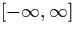Next: Transcendental Functions Up: High-Level Operations Previous: Recursive calls to compute

# Limits withinSection 5.2 shows how we can convert a stream of nested intervals in the range [-1,1] into a signed binary stream. It would be more useful to be able to convert streams of nested intervals from the whole real line.

The problem here is that using the (mantissa, exponent) representation for end-points, the numeral for each end-point can have different a exponent and we cannot use the mantissa's directly as we could in section 5.2. Fortunately, we can easily choose an exponent with which can represent the final output, and all other end-points in the stream of nested intervals, by simply taking the maximum exponent required at the first interval of the stream. Because the intervals are strictly nested, we can then re-represent all subsequent end-points using this exponent and operate solely on the mantissas using the previous method, and outputting a result using the chosen exponent.

Martin Escardo
5/11/2000